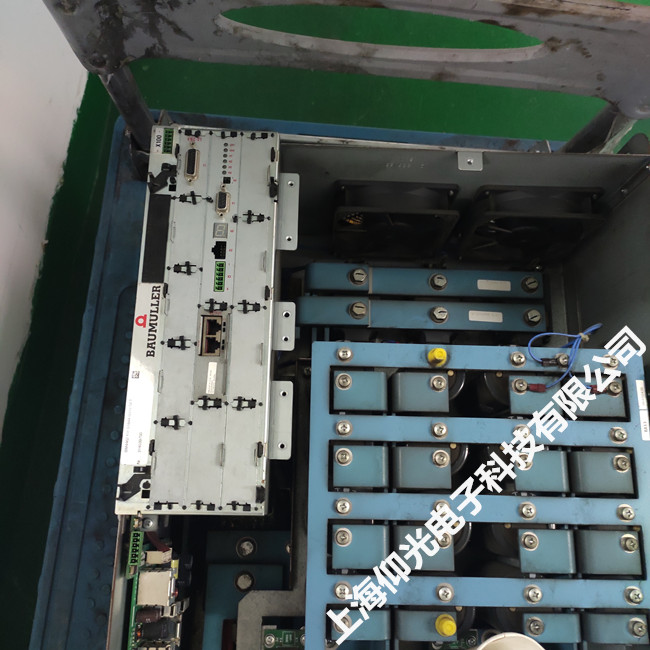Hi，欢迎访问上海仰光电子科技有限公司！

## 上海包米勒Baumuller bm4400驱动器故障报警解析P0207

96 =短路温度传感器（Tm <= -30°C）
97 =温度传感器 - 未连接电机（Tm> + 300°C）
98 =电机温度过高
99 =错误I2t> 100％
100 =功率单元最大电流>电机最大电流101保留
102=集体错误发现缺口位置
103-111=保留

P0208

112=通信错误（HIPERFACE规范）
113=保留
114=覆盖编码器位置信息时出错
115=断线编码器1
116=超速编码器1
117 =超出幅度限制
118 =编码器类型未知
119 =电机数据的数据字段无效
120 =电机数据不正确
121 =保存电机数据的错误
122 =电机数据写保护。（对BM电机无效）
123 =场角误差
124 =没有温度测量的编码器
125 =编码器电子版中的存储容量太小
126-127=保留

P0209

128 =通信错误（HIPERFACE规范）
129保留
130 =覆盖编码器位置信息时出错
131 =断线编码器2
132 =超速编码器2
133 =超出幅度限制
134 =编码器类型未知
135 =电机数据的无效数据字段
136 =电机数据不正确
137 =保存电机数据的错误
138 =电机数据写保护。（对BM电机无效）139=Field angle error
140 =没有温度测量的编码器
142-143保留
P210

144 =编码器1未知的绝对位置
145 =编码器2未知的绝对位置
146 =缺少编码器模块1
147 =缺少编码器模块2
148 =缺少测量值存储的编码器模块
149 =在解析器中没有测量值存储可能
150 =触发不可能，因为没有增量编码器
151 =缺少数字I / O模块
152 =增量编码器仿真模块是必需的并且缺失
153 =编码器模块1是增量编码器仿真和输出所必需的
154 =编码器模块2是增量编码器仿真和输出所必需的
155 = 增量编码器仿真模块的初始化错误
156 =增量编码器仿真模块（HW）发出错误信号
157 =错误增量编码器仿真模块
158 = SSI编码器仿真模块正在丢失
159 =设定值源编码器1或2出错

P211

160 =超时通信
161 = BACI通信选项模块的超时
162 =超时循环通信
163 =超时所需数据
164 =现场总线错误
165 =控制器与外部信号不同步
166 =制动控制错误
167 =启动驱动器时没有松开抱闸
168 =停止驱动时没有关闭抱闸
169 =保持制动状态错误（循环监控）170 =保持制动衬片错误
171 =初始化抱闸错误
172 =抱闸错误：保持转矩不准
173-175=保留

P212

176 = EEPROM复制错误
177 =写入超时EEPROM
178 =校验和错误EEPROM
179 =没有启动数据集
180 =不兼容的软件
181 =没有数据集
182 = PSI模块的校验和错误
183 = PSI已重置请保存所有数据记录
184 = PSI数据无效
185 =自动调整表无效
186 = A / D校正表无效
187 = EEPROM复位
188-191=保留

P213

192=位置动态偏差
193=位置静态偏差
194=编码器1用于位置控制，但处于非活动状态。如果故障定位位于其中一个非活动数据线中，也会显示此错误
195=编码器2用于位置控制，但处于非活动状态。如果故障定位位于其中一个非活动数据线中，也会显示此错误。
196=软件限位开关1超出
197=超出软件限位开关2
198=硬件限位开关1超出可调节性
199=硬件限位开关2超出可调节性
200=返回未执行
201=模式设定点设定点没有及时到达
202=最小化目标位置或调整模数位置P1239
203=主轴定位：触发器初始化时出错
204=主轴定位：触发信号超时
205=执行归位时发生错误
206-207保留

【 我们确保修好测试好给客户！！！！】